Splitting Pixels

By angros47

Usually, the smallest thing that can be drawn on your screen is a single pixel; actually, it's not always true. In this tutorial, we'll learn how to use only part of a pixel, to gain an higher resolution.

First, we need to understand how pixels are made: a combination of red, green and blue is used to produce all the other colors …. so, on a color display a pixel needs to provide a source of red, green and blue light.

Different kinds of display can use a different solution: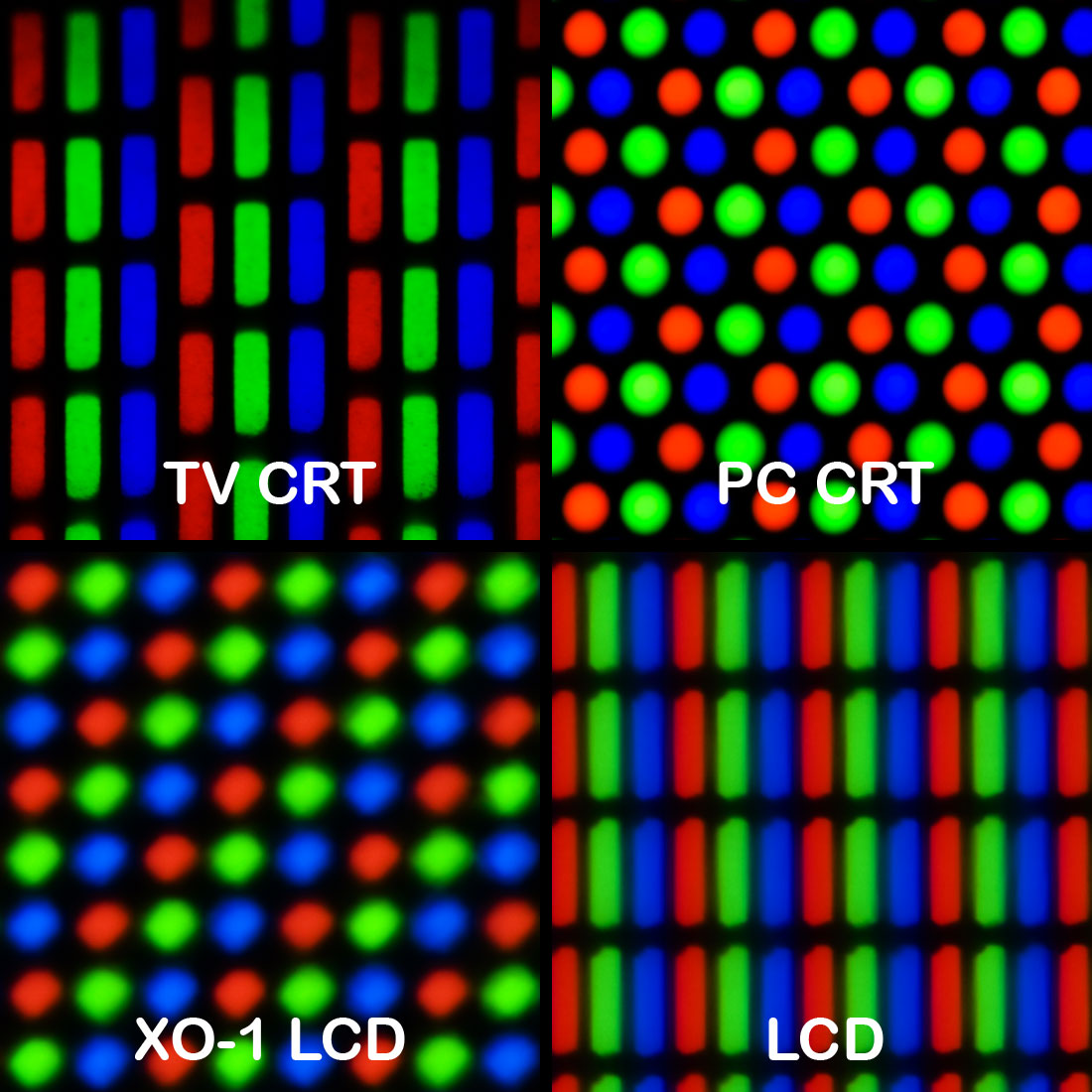on a LCD monitor, usually a pixel is made by three rectangles, of three colors:

This means that, in the same pixel, the red section is on the left, the green one is in the middle, the blue one is on the right.

This could lead to some artifacts on the final image: let's have a look: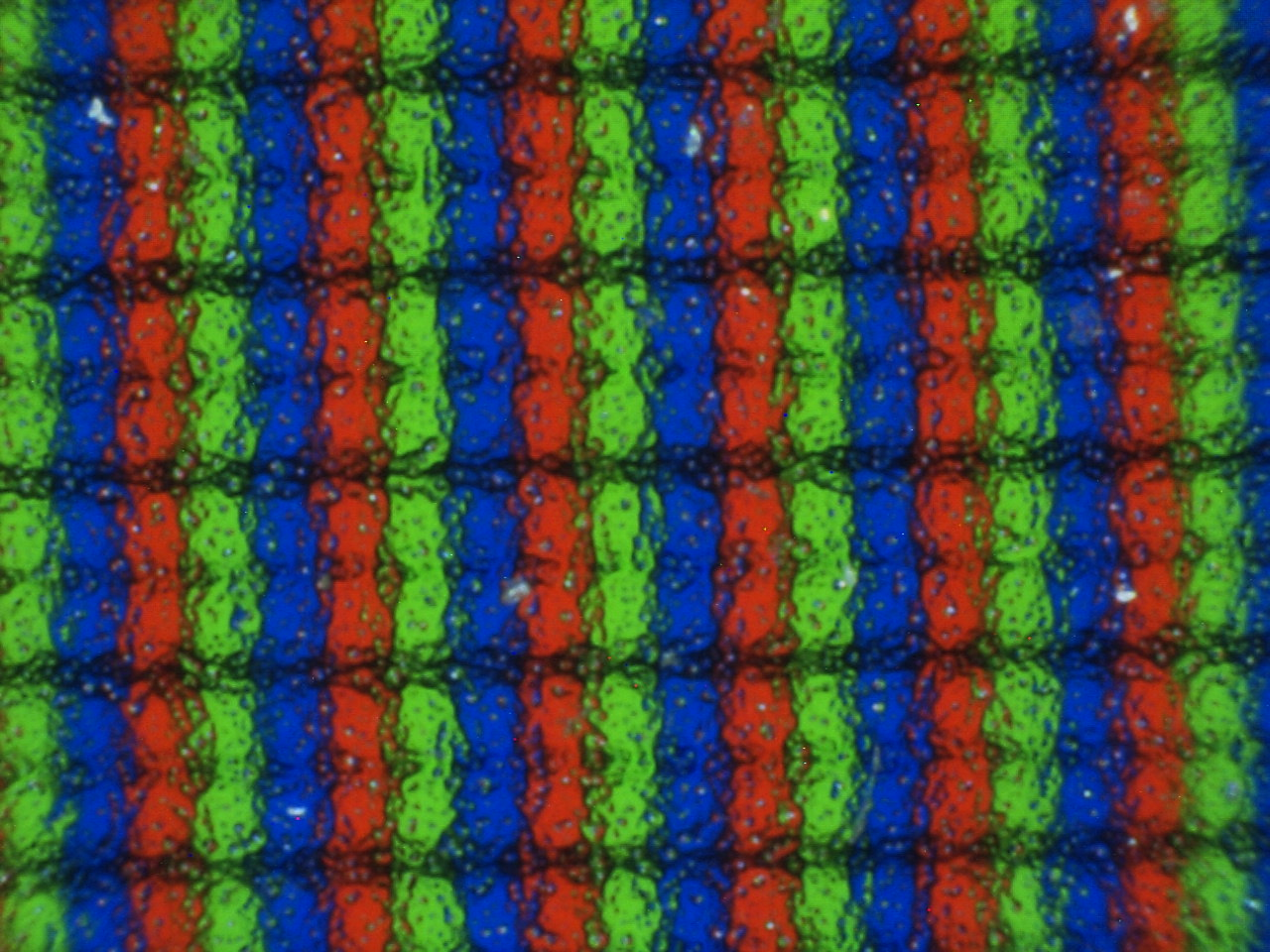Warning: all the examples are expected to work on a LCD display, at the maximum resolution (a pixel drawn in FreeBasic is a pixel on the screen); in windowed mode, this usually is not a problem, but in full-screen mode (i.e. in DOS), unless you set the maximum resolution available, a point is made using more than one pixel, so the example won't work.

A first example could be:

screenres 640,480,32
line (0,240)-(640,240),rgb(255,0,255)
line (320,0)-(320,480),rgb(255,0,255)
sleep

It will draw an horizontal and a vertical line, in color magenta.
But... try looking closer: the horizontal line is right, but do you see a red line at the left of the vertical line?

Maybe you can see it better in another example:

screenres 640,480,32
line(320,0)-(320,240),rgb(255,0,255)
line(320,240)-(320,480),rgb(0,255,0)
sleep

See that the upper line is thicker than the lower line?

To understand what happened, let's use a microscope:

The horizontal line: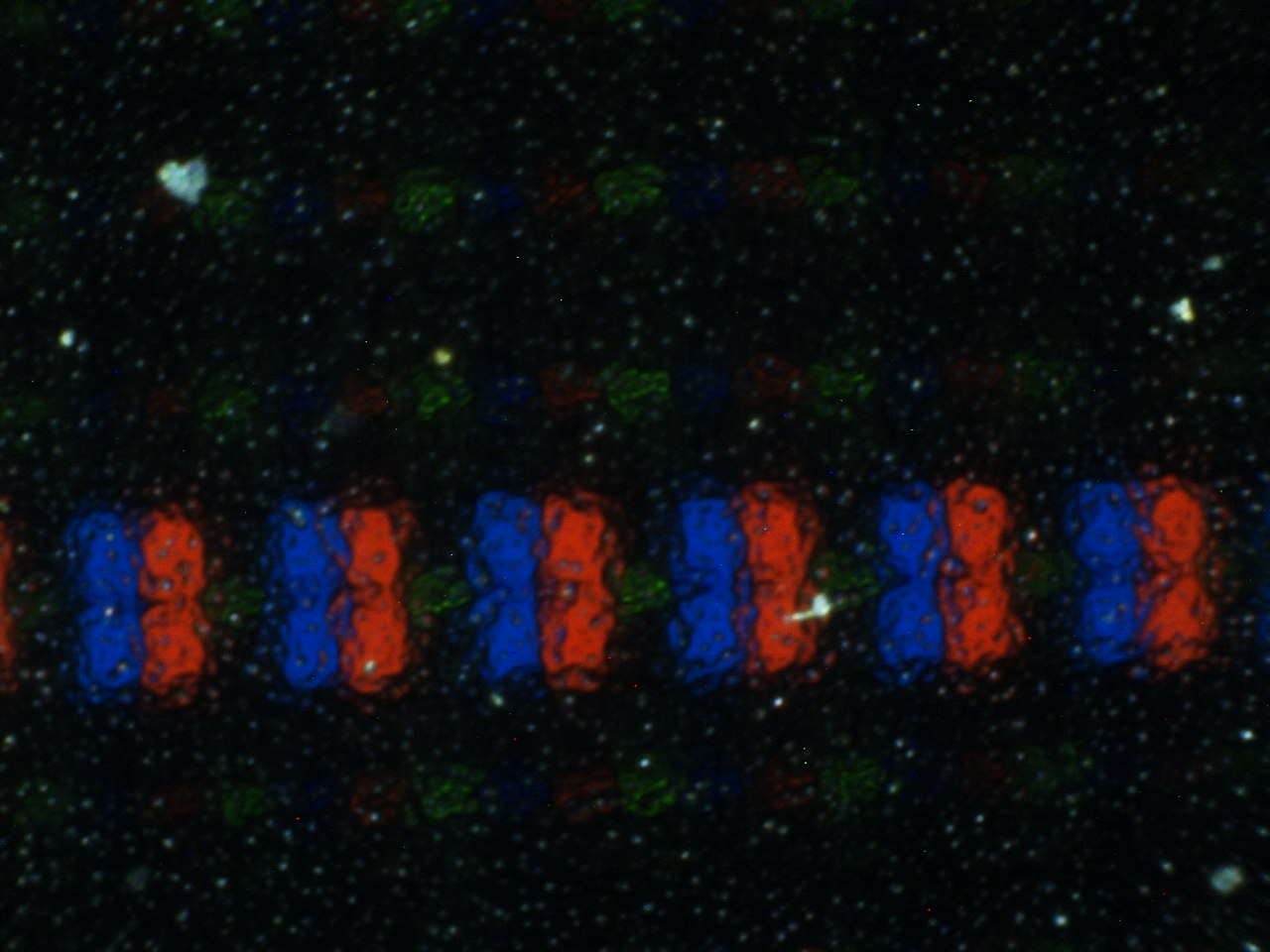The vertical line: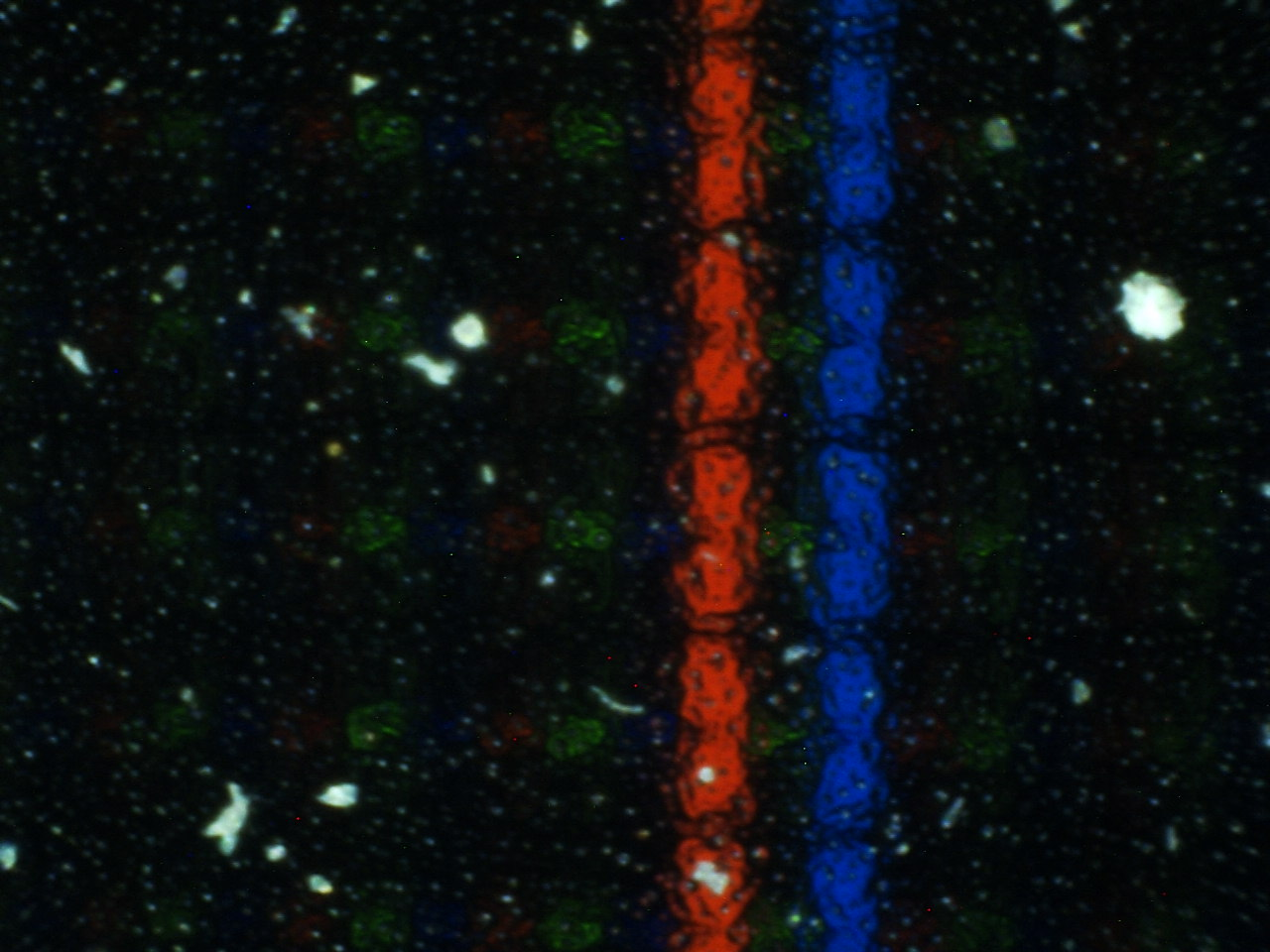And... here is the green line: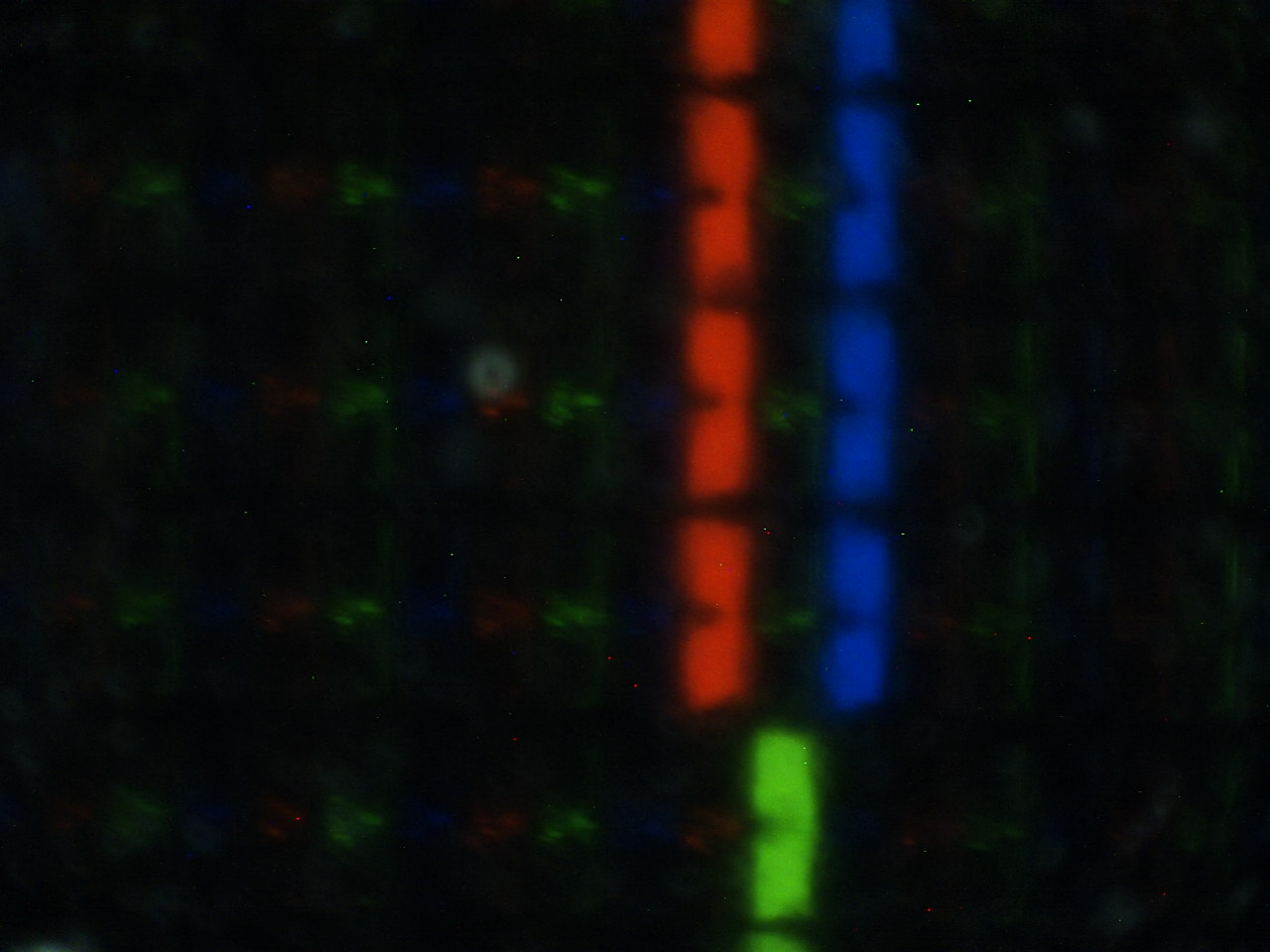When we use two pixels, artifacts are more evident:

screenres 640,480,32
line (0,240)-(640,240),rgb(255,0,0)
line (0,241)-(640,241),rgb(0,0,255)
line (320,0)-(320,480),rgb(255,0,0)
line (321,0)-(321,480),rgb(0,0,255)
sleep

Do you see? We draw two adjacent lines (one red, one blue), but between the vertical lines there is a black line! The horizontal lines are correctly displayed, again.

In fact, under the microscope, the horizontal lines are: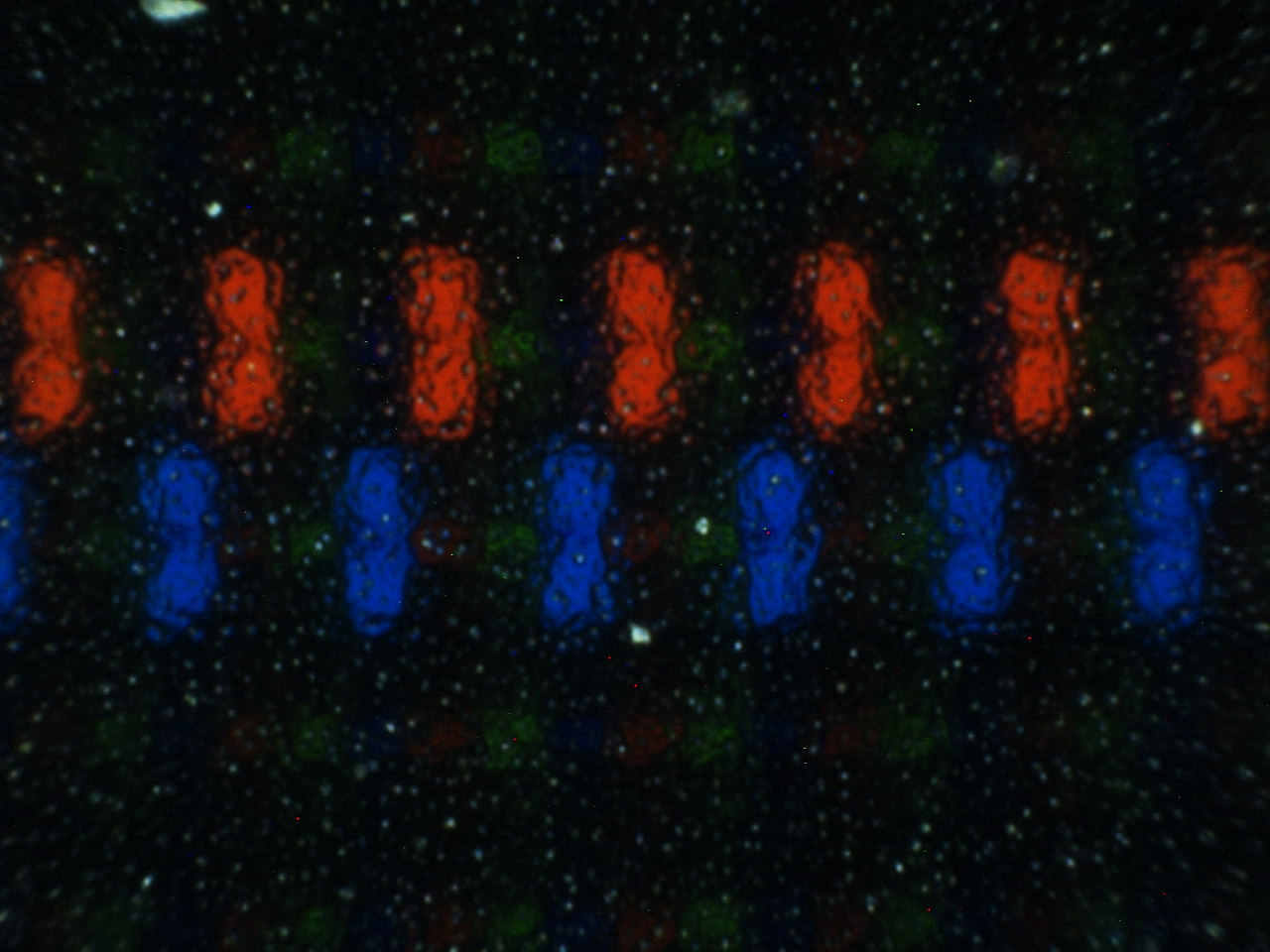while the vertical lines are:Ok, so we've just learned that pixels can be splitted: in fact, we have deliberately chosen areas that are distant, inside pixels. But we can also do the opposite, merging adjacent pixels.

Let's just reverse the last example:

screenres 640,480,32
line (0,240)-(640,240),rgb(0,0,255)
line (0,241)-(640,241),rgb(255,0,0)
line (320,0)-(320,480),rgb(0,0,255)
line (321,0)-(321,480),rgb(255,0,0)
sleep

We have just swapped the colors, nothing more: as usually, the horizontal lines are right, but what happened to vertical lines? There is only one line, and it's neither red, nor blue, but magenta!

Our trusty microscope comes to the rescue:
nothing of interest in the horizontal lines...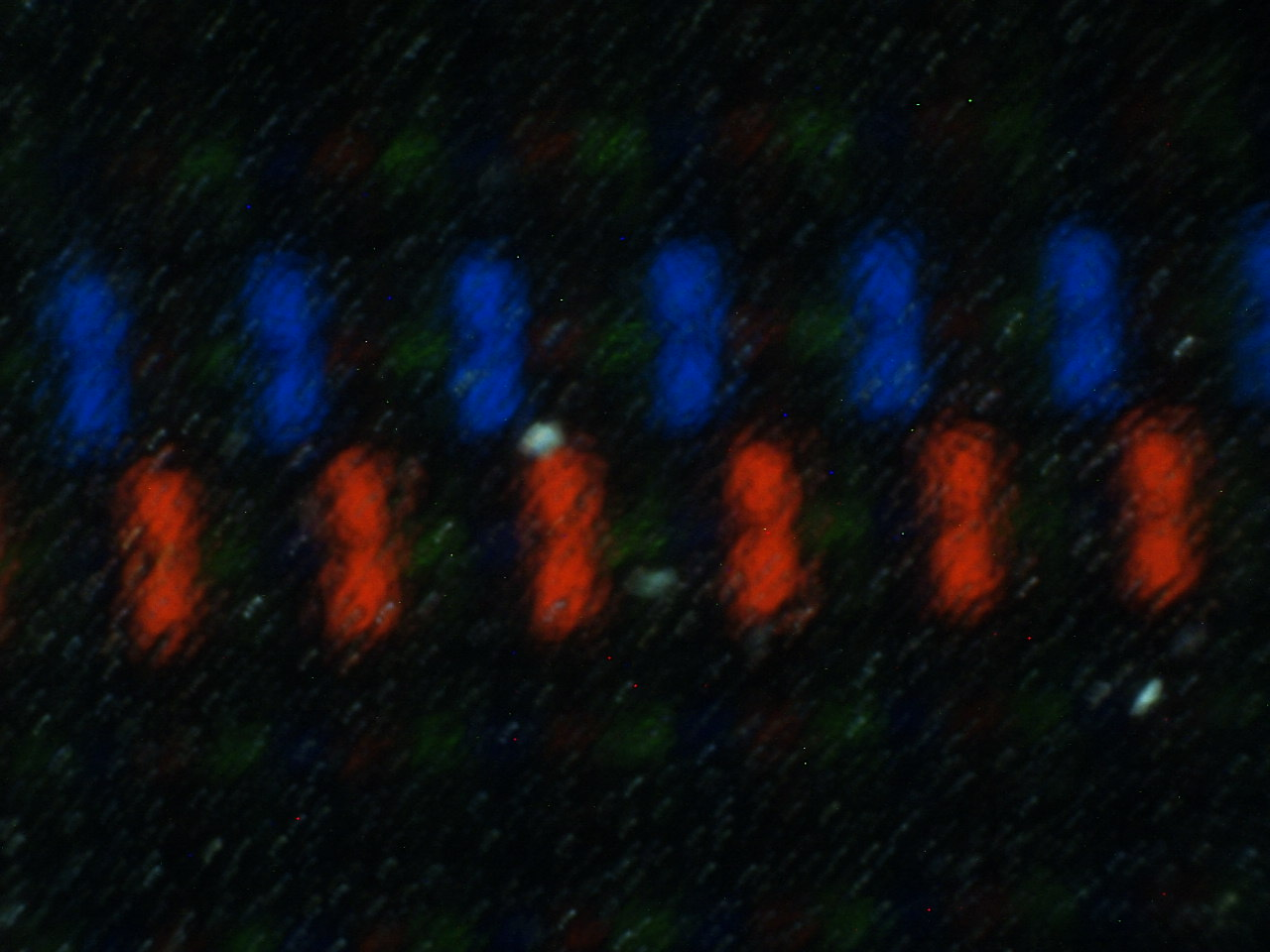… but, on vertical lines, it finally unveils the secret:red and blue elements of two different pixels are too close, so we see only one point: and since red and blue give magenta, we see a magenta pixel.

Magenta is the only colour we can get? Of course not! We can get white, for example:

screenres 640,480,32
line (0,240)-(640,240),rgb(0,255,255)
line (0,241)-(640,241),rgb(255,0,0)
line (320,0)-(320,480),rgb(0,255,255)
line (321,0)-(321,480),rgb(255,0,0)
sleep

Cyan and red lines can give us a vertical white line.

On the microscope: horizontal lines...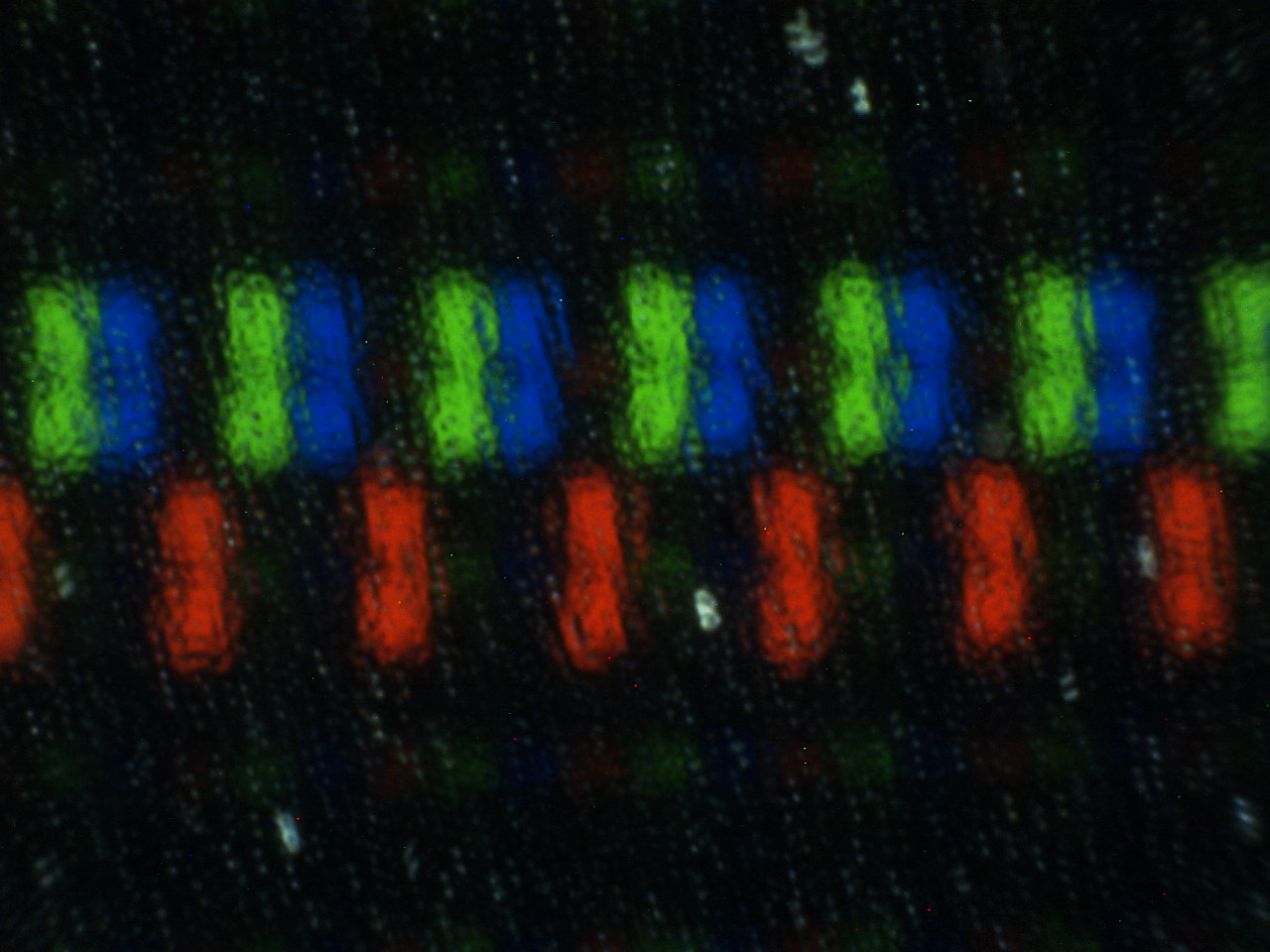… and vertical line: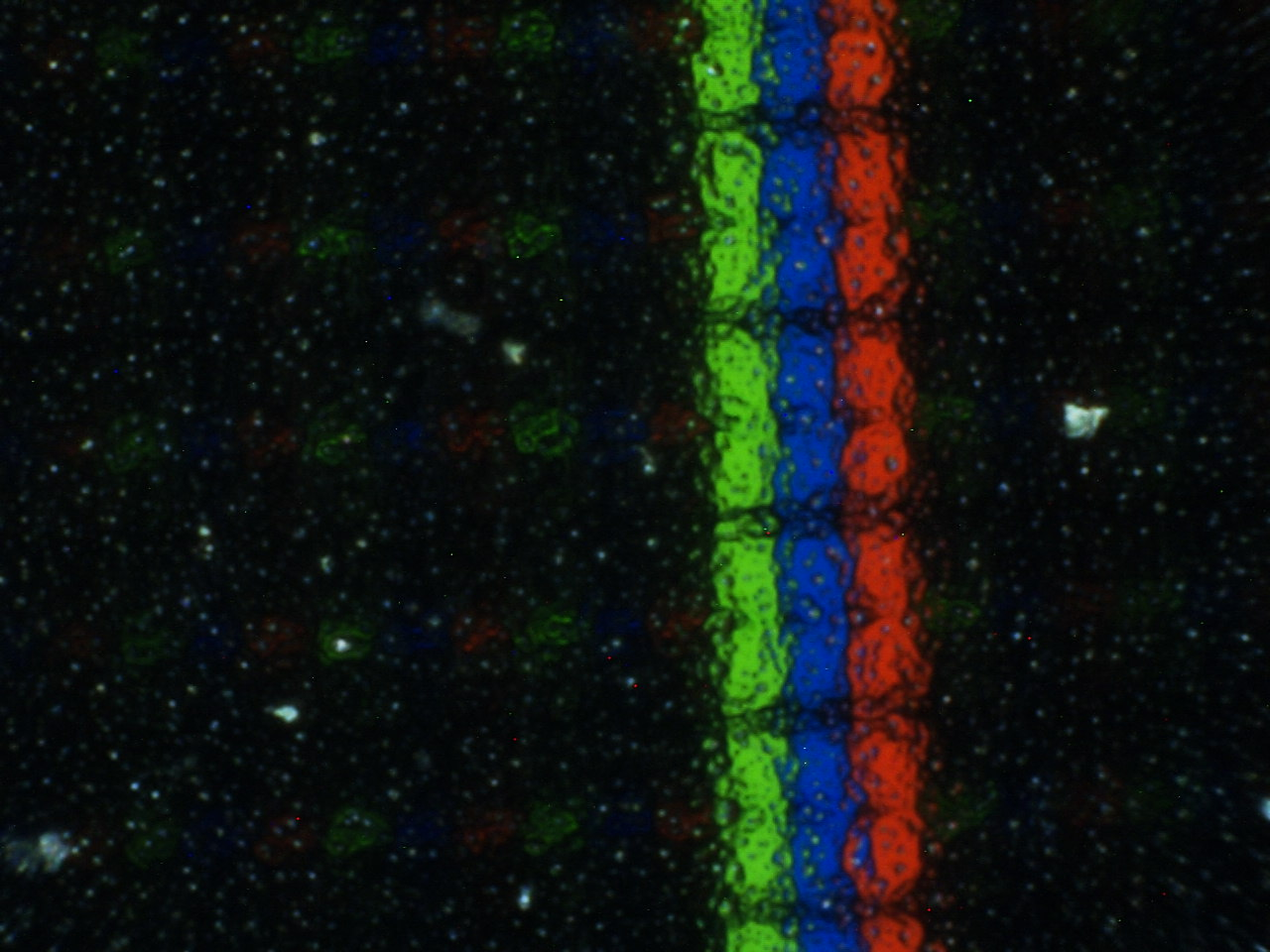As we can see, we have red, green and blue elements... therefore we get a square, white pixel! But this pixel is shifted to the right by a third of pixel width (in fact, its red element, that is a third of the pixel, is off, while the red element of the adjacent pixel, on the right, is on)

To prevent artifacts, many rendering engines use an anti-alias filter (on last versions of OSX, the anti-alias is active by default); but these artifacts can also be used to increase video resolution (it's not a new idea: the first computer that used a similar trick was the Apple II)

In FreeBasic, for example, we can do:

screenres 640,480,32
pset (320,240),rgb(255,255,255) ' First pixel
pset (321,240),rgb(0,0,0)
sleep
pset (320,241),rgb(0,255,255) ' Second pixel: shifted by one-third
pset (321,241),rgb(255,0,0)
sleep
pset (320,242),rgb(0,0,255)   ' Third pixel: shifted by two-thirds
pset (321,242),rgb(255,255,0)
sleep
pset (320,243),rgb(0,0,0)
pset (321,243),rgb(255,255,255) 'Fourth pixel: normal, again
sleep
sleep

The result is something like: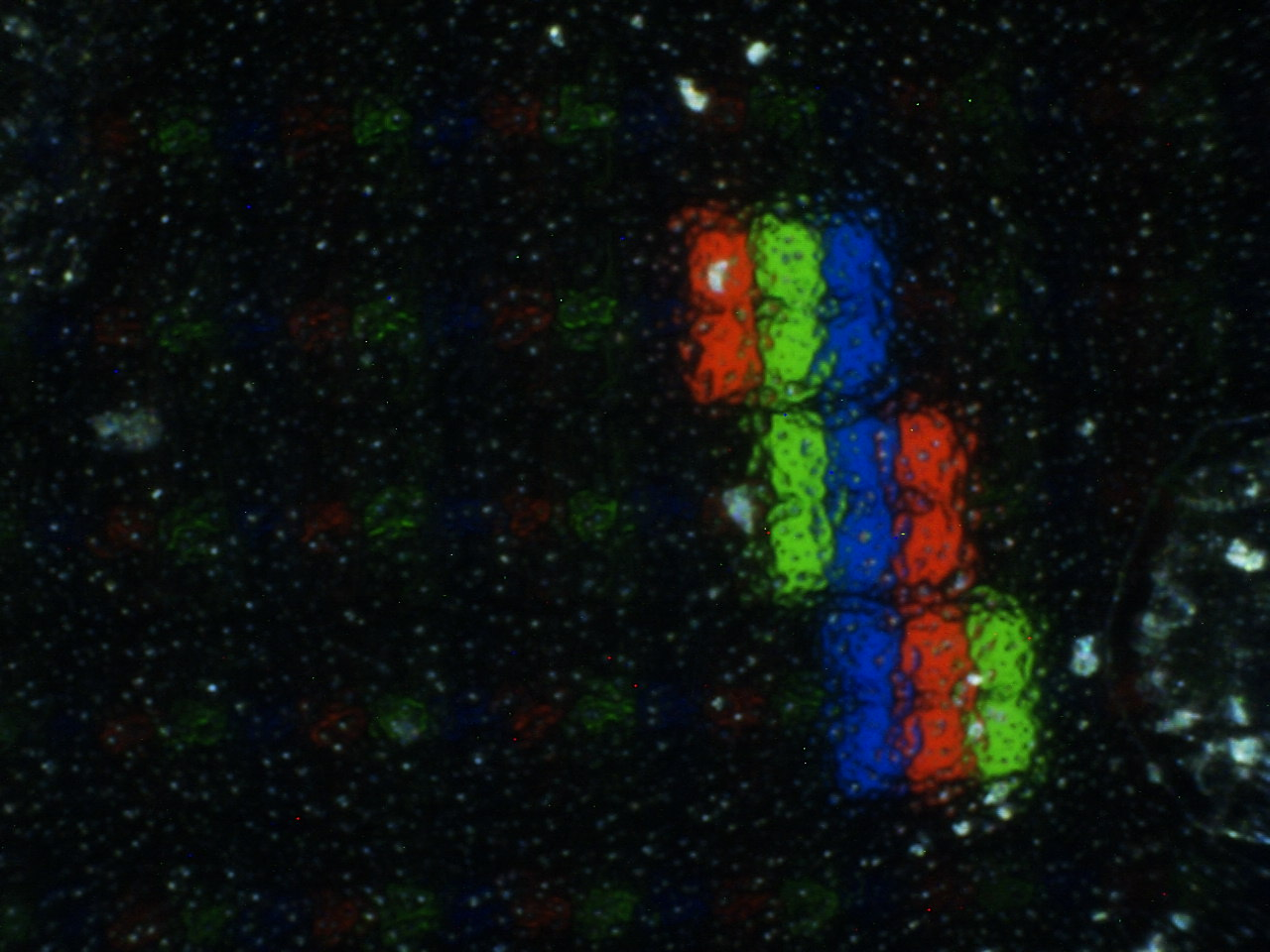Some font rendering engines can use a similar trick to draw smooth characters.

One last note: this trick relies on how LCD are built; if, in the future, displays will be built in another way, this solution won't work anymore: so, don't assume that the hardware will always support it, and provide an option to disable it.